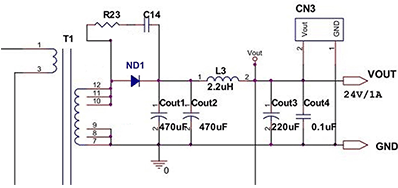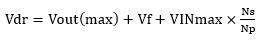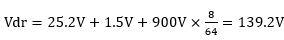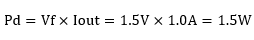Technical Information Site of Power Supply Design

• Basic Knowledge
• AC/DC
• Design Example of Isolated Quasi-Resonant Converters Using SiC MOSFET
• Selecting Critical Components: Output Rectifying Diode

2019.03.07 AC/DC

# Selecting Critical Components: Output Rectifying Diode

Design Example of Isolated Quasi-Resonant Converters Using SiC MOSFET

In this article, we calculate the component values of the diode used for DC rectification of the switching voltage on the secondary side (output).

Output Rectifying Diode：ND1

We begin by explaining the circuit diagram. The output rectifying diode ND1 is the diode with the dark blue color in the circuit diagram on the right.

Because the output rectifying diode rectifies a switching voltage, a fast diode (Schottky barrier diode, fast recovery diode) is used.First we calculate the reverse voltage applied to the output diode.If we assume that Vf＝1.5V and Vout (max)＝24.0V+5%＝25.2V, then we obtainfor the reverse voltage.

Setting a 30% margin, we select a component that has a rated voltage of 200 V (139.2 V/0.7 = 198 V → 200 V).

As a rough estimate of the diode loss, we calculate as follows.As a diode satisfying these values, we select the ROHM fast recovery diode RFN10T2D (common cathode dual type, 200 V/10 A, TO-220FN package).

In essence, it is desirable that the diode be used at 70% or lower of the rated voltage and at 50% or lower of the rated current. Actual temperature increases should be checked with the components incorporated into the product, and when necessary, heat sinks should be added, or components should be re-evaluated.

#### Key Points:

・As output rectifying diodes, fast recovery diodes or Schottky barrier diodes are used.

・Diodes should be selected such that the device is used within 70% of the rated voltage and within 50% of the rated current.